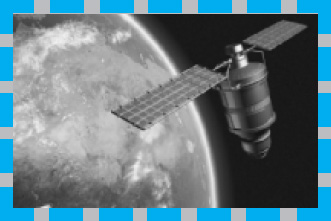# Systems of Two First Order EquationsThe first two chapters of this book contain the material most essential for dealing with single first order differential equations. To proceed further, there are two natural paths that we might follow. The first is to take up the study of second order equations and the other is to consider systems of two first order equations. There are many important problems in various areas of application that lead to each of these types of problems, so both are important and we will eventually discuss both. They are also closely related to each other, as we will show. Our approach is to introduce systems of two first order equations in this chapter and to take up second order equations in Chapter 4.

There are many problem areas that involve several components linked together in some way. For example, electrical networks have this character, as do some problems in mechanics and in other fields. In these and similar cases, there are two (or more) dependent variables and the corresponding mathematical problem consists of a system of two (or more) differential equations, which can always be written as a system of first order equations. In this chapter, we consider ...

Get Differential Equations: An Introduction to Modern Methods and Applications, 2nd Edition now with the O’Reilly learning platform.

O’Reilly members experience live online training, plus books, videos, and digital content from nearly 200 publishers.